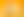The Earth in Space: Passing Time

# Stages1. Awareness of Time

EXPLORE

Suggested Learning Intentions

• To understand that the passage of time is measured in specific units based on the movement of the Earth in space

Sample Success Criteria

• I can demonstrate that the passage of a day is determined by the length of time that it takes for the Earth to spin on its axis
• I can demonstrate that the passage of a year is determined by the earth’s movement around the sun, which takes approximately 365 days
• I can explain why we have leap years
• I can demonstrate that seasons are determined by the Earth’s orbit around the sun and the earth’s relative axial tilt
• I can describe that the passage of a month is determined by one cycle of the moon’s phases
• I can use manipulatives to demonstrate my understanding
EXPLORE

2. Measurement of Time

EXPLORE

Suggested Learning Intentions

• To understand that measuring devices measure the passage of time
• To understand the structure and operation of time measuring devices
• To understand the relationships between units of time so that time can be measured accurately

Sample Success Criteria

• I can explain how different measuring devices measure different units of time
• I can describe the difference between an analogue clock and a digital clock
• I can demonstrate the relationship between different units of time
• I can choose an appropriate unit of time to calculate elapsed time depending upon what I am calculating
• I can accurately compare the duration or succession of various events by converting between years, months, and days
EXPLORE

3. Succession of Time

EXPLORE

Suggested Learning Intentions

• To understand that two or more different events are organised sequentially, and that events can occur simultaneously
• To understand that successive events are marked by the iteration (repetition) of the duration of various units of time

Sample Success Criteria

• I can sequence a series of events in order based on when they occurred or will occur
• I can list events that occur at the same time
• I can create, interpret, and follow a timetable using successive time slots
• I can describe succession of time in hours and minutes
• I can create a timeline of events involving past, present, and future
• I can demonstrate my understanding using manipulatives
EXPLORE

4. Duration of Time

EXPLORE

Suggested Learning Intentions

• To understand that elapsed time is the duration of an event from its beginning to its end
• To understand that the duration of an event can be measured in unbroken units of time from the very small to the very large

Sample Success Criteria

• I can work out the length of an event by comparing the start and the end time of the event
• I can work out the length of an event in a variety of ways, such as skip counting using an analogue clock or jumping forwards or backwards along an empty number line
• I can work out the duration of an event by converting units of time when needed
• I can use manipulatives to explain my thinking and problem solving
EXPLORE